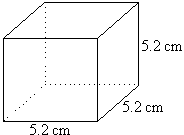## How to Calculate and Solve for the Centre of Gravity of a CubeThe image above is a cube with a length of 5.2 cm.
To compute the centre of gravity of a cube, one essential parameter is needed and this parameter is the length of the cube (l).

The formula for calculating the centre of gravity of a cube is:

C.G. = 0.5(l)

Where:
l = Length of the Cube
C.G. = Centre of Gravity

Let’s solve an example:
Find the centre of gravity of a cube where the length of a side of the cube is 5.2 cm.

This implies that:
l = Length of the Cube = 5.2